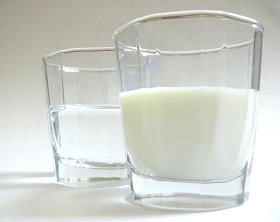# Be careful. It's careless

Algebra Level 3There are two containers named $A$ and $B$. Container $A$ has milk in it, container $B$ has water (same amount as of milk in container $A$) in it. Some amount of milk is transferred from container $A$ to container $B$ and then , the same amount is transferred from the container $B$ to the container $A$.

Let container $A$ has $x$ amount of water in it and container $B$ has $y$ amount of milk in it.

Which one option is correct?

Note : Finally the amount in both the containers is same as it was initially.

×

Problem Loading...

Note Loading...

Set Loading...